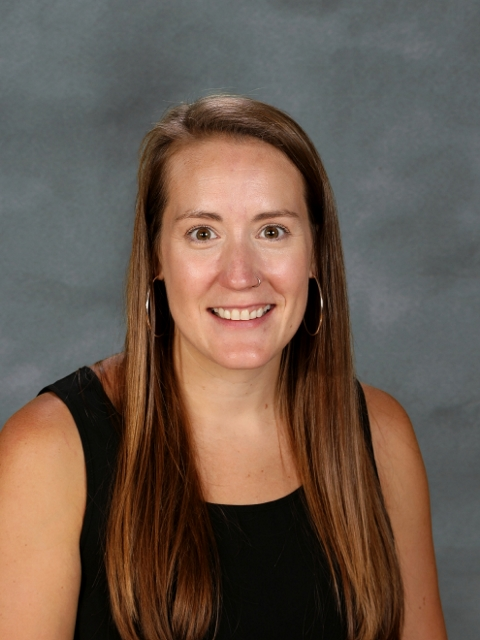#### Mathematics

The CHS Math Department creates an environment that cultivates mathematical reasoning and meets students at their current level of understanding, so all learners can grow and develop their knowledge and skills. We do this by providing multiple opportunities for learning, and by encouraging students to think flexibly and solve problems in a variety of ways. Our teaching methods stimulate curiosity, encourage persistence, and incorporate technology. We encourage students to be self-sufficient learners who know when to use tools and resources as they model and analyze problems. CHS graduates will achieve a level of math literacy appropriate to any future path they choose.
Mission Statement

#### Proficencies

1. Model: The CHS graduate can use mathematics to explore the relationships among quantities in context and use these relationships to draw conclusions.
2. Problem Solve: The CHS graduate can persist in solving a problem independently by analyzing, making predictions, and using mathematical methods to develop a reasonable solution.
3. Algebra & Functions: The CHS graduate can create, interpret, use and analyze expressions, equations, inequalities, and functions in a variety of contexts.
4. Statistics & Probability: The CHS graduate can interpret and apply statistics and probability to analyze data, reach and justify conclusions, and make inferences.
5. Geometry: The CHS graduate understands geometric concepts and constructions and can use them to prove theorems and to solve a variety of problems.Jaclyn Dixon